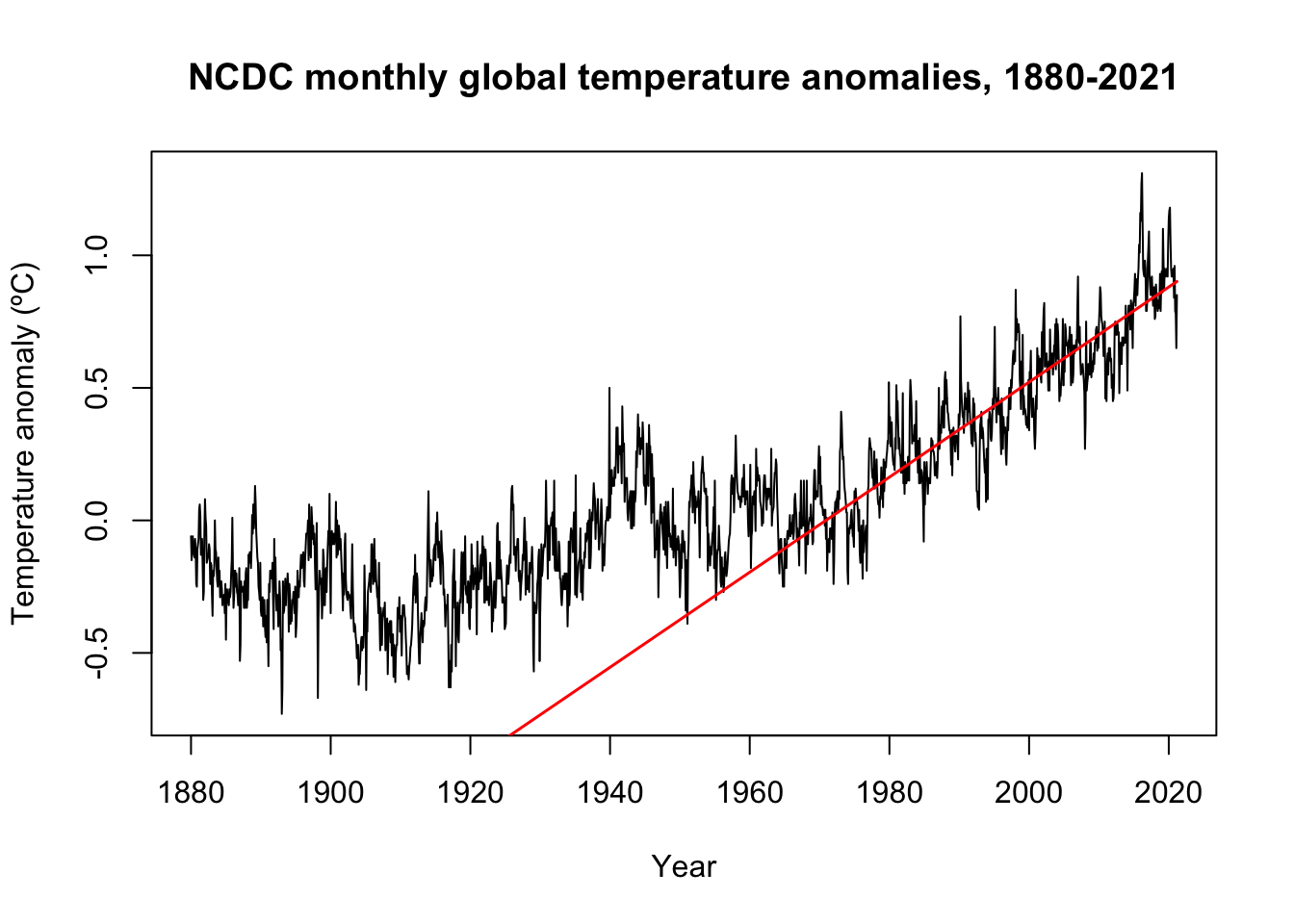## Introduction

This is a repeat of an analysis I first did in 2013. One of the signatures of climate change is that it alters the frequency and probability of climate-related events like the global monthly mean temperature. Let me illustrate.

### Temperature Data

Let’s use the NOAA NCDC data for this analysis. You can get it online at https://www.ncdc.noaa.gov/cag/global/time-series/globe/land_ocean/1/2/1880-2021/data.csv.

``````URL <- "https://www.ncdc.noaa.gov/cag/global/time-series/globe/land_ocean/all/1/1880-2021/data.csv"
setwd("~/Desktop/Climate")
noaa <- noaa[-c(1:5), ]
names(noaa) <- c("Year", "Temperature")
noaa\$Time <- seq.Date(as.Date("1880-01-01"), origin = "1970-01-01", by = "month", length.out = length(noaa\$Temperature))
noaa\$Temperature <- as.numeric(noaa\$Temperature)``````

Once we have the data formatted, we can do an analysis like this for starters: I created a subset of the data, then used linear regression to find the trend since 1970.

``````noaa.ts <- ts(noaa\$Temperature, start = c(1880, 1), frequency = 12)
noaa_1970 <- subset(noaa, Time >= as.Date("1970-01-01"))
noaa.1970.ts <- ts(noaa_1970\$Temperature, start = c(1970, 1), frequency = 12)
plot(noaa.ts, xlab = "Year", ylab = "Temperature anomaly (ºC)", main = "NCDC monthly global temperature anomalies, 1880-2021")
noaa_arima <- auto.arima(noaa.1970.ts, ic = c("bic"))
n_a_order <- arimaorder(noaa_arima)
noaa_gls <- gls(noaa.1970.ts~time(noaa.1970.ts), correlation = corARMA(p = n_a_order, q = n_a_order))
curve(noaa_gls\$coefficients + noaa_gls\$coefficients*x, add = TRUE, col = "red", lwd = 1.5)````````noaa_ci <- intervals(noaa_gls)``

This is the familiar way to show that mean global temperatures have risen over time. Since 1970, global temperatures have risen 0.1793431 per decade (95% confidence interval 0.1707054 to 0.1879808). However, it doesn’t really help the reader intuitively grasp just how that rise changes the probability of certain monthly mean temperatures. For that, we can calculate the density kernel of different decades.

``````#Split the temperature record into different decades
noaa.1880_1889 <- subset(noaa, Time >= as.Date("1880-01-01") & Time < as.Date("1890-01-01"))
noaa.1950_1959 <- subset(noaa, Time >= as.Date("1950-01-01") & Time < as.Date("1960-01-01"))
noaa.1970_1979 <- subset(noaa, Time >= as.Date("1970-01-01") & Time < as.Date("1980-01-01"))
noaa.1980_1989 <- subset(noaa, Time >= as.Date("1980-01-01") & Time < as.Date("1990-01-01"))
noaa.1990_1999 <- subset(noaa, Time >= as.Date("1990-01-01") & Time < as.Date("2000-01-01"))
noaa.2000_2009 <- subset(noaa, Time >= as.Date("2000-01-01") & Time < as.Date("2010-01-01"))
noaa.2010_2019 <- subset(noaa, Time >= as.Date("2010-01-01") & Time < as.Date("2020-01-01"))

#Create the density kernels
D1880s <- density(noaa.1880_1889\$Temperature)
D1950s <- density(noaa.1950_1959\$Temperature)
D1970s <- density(noaa.1970_1979\$Temperature)
D1980s <- density(noaa.1980_1989\$Temperature)
D1990s <- density(noaa.1990_1999\$Temperature)
D2000s <- density(noaa.2000_2009\$Temperature)
D2010s <- density(noaa.2010_2019\$Temperature)

#Graphing the density kernels
plot(D1880s, main="Density plot of global temperatures during selected decades", xlab="Temperature anomalies (ºC)",xlim=c(-0.75,1.5), ylim=c(0,4), lwd=1.5)
points(D1950s, type = "l", col = "blue", lwd = 1.5)
points(D1970s, type = "l", col = "darkgreen", lwd = 1.5)
points(D1980s, type = "l", col = "green", lwd = 1.5)
points(D1990s, type = "l", col = "orange", lwd = 1.5)
points(D2000s, type = "l", col = "darkred", lwd = 1.5)
points(D2010s, type = "l", col = "red", lwd = 1.5)
legend("topleft", legend = c("1880s", "1950s", "1970s", "1980s", "1990s", "2000s", "2010s"), col = c("black", "blue", "darkgreen", "green", "orange", "darkred", "red"), lwd = 2)``````